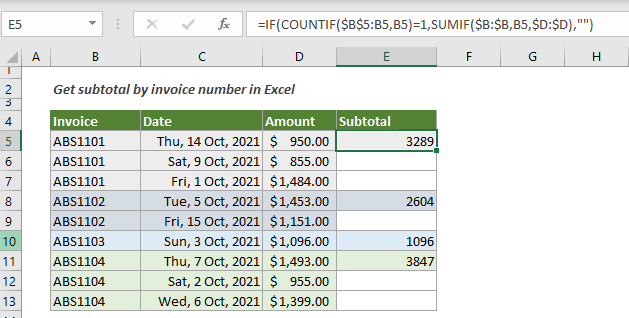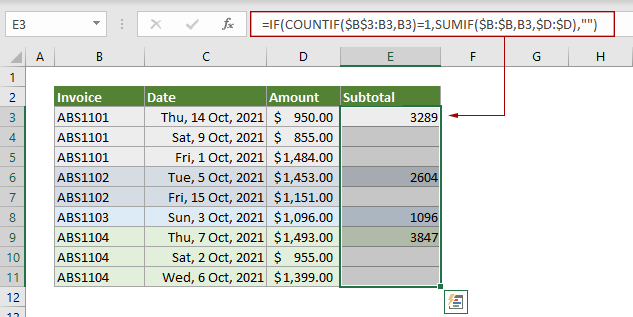## Get subtotal by invoice number in Excel

This tutorial explains a formula based on the COUNTIF and SUMIF functions to get subtotal by invoice number in Excel.#### How to get subtotal by invoice number in Excel?

Take the above table as an example, to get subtotal by the first occurrence of the invoice number, you can apply the below formula.

Generic Formulas

=IF(COUNTIF(range,criteria)=1,SUMIF(range,criteria,sumrange,"")

Arguments

Range: Represents the expanding range of the invoice number that you want to get subtotal based on;
Criteria: Represents the first invoice number. It can be a text string wrapped in double quotation marks, or a cell that references the invoice number;
Sumrange: Represents the range that you will sum.

How to use this formula?

1. Firstly, select a blank cell to output the result. In this case, I select a blank cell (E5) in the same row as the first invoice number.

2. Copy or enter the below formula into the selected cell and press Enter key. Select the result cell, drag its AutoFill Handle down to get the subtotals for other invoice numbers.

=IF(COUNTIF(\$B\$3:B3,B3)=1,SUMIF(\$B:\$B,B3,\$D:\$D),"")Explanation of this formula

=IF(COUNTIF(\$B\$3:B3,B3)=1,SUMIF(\$B:\$B,B3,\$D:\$D),"")

> COUNTIF(\$B\$3:B3,B3)=1: Here the COUNTIF function is used with an expanding range to check if the current row is the first occurrence of a given invoice number. If it is, it returns TRUE, otherwise it returns FALSE.
Here, in this case, ABS1101 in cell B3 is the first occurrence of the invoice number of ABS1101, then it gets the result of COUNTIF(1=1): TRUE.
> SUMIF(\$B:\$B,B3,\$D:\$D)The SUMIF function performs a total sum in the Subtotal column based on the invoice number. Here the SUMIF function returns the result of 3289.
> IF(TRUE,3289,"")If the result obtained by the COUNTIF function is TRUE, the SUMIF function will return the result of this calculation, otherwise, it will return a null value. The final result here is 3289.

#### Related functions

Excel IF function
The Excel IF function performs a simple logical test which depending on the comparison result, and it returns one value if a result is TRUE, or another value if result is FALSE.

Excel COUNTIF function
The Excel IF function is a statistical function in Excel which is used to count the number of cells that meet a criterion.

Excel SUMIF function
The Excel SUMIF function can help to sum cells based on one criterion

#### Related formulas

3D sum or sumif across multiple worksheets
This tutorial talks about how to sum the same range of cells across multiple consecutive or discontiguous worksheets in Excel.

Calculate running total
This tutorial explains a simple formula to help calculating running total in Excel.

Subtotal by colors
This step-by-step guide helps you perform subtotal by color with the SUMIF function.

Two-way summary counting
In this tutorial, we will discuss how to use the COUNTIFS function to create two-way summary count in Excel.

### The Best Office Productivity Tools

#### Kutools for Excel - Helps You To Stand Out From Crowd

 Popular Features: Find/Highlight/Identify Duplicates  |  Delete Blank Rows  |  Combine Columns or Cells without Losing Data  |  Round without Formula ... Super VLookup: Multiple Criteria  |  Multiple Value   |  Across Multi-Sheets  |  Fuzzy Lookup... Adv. Drop-down List: Easy Drop Down List  |  Dependent Drop Down List  |  Multi-select Drop Down List... Column Manager: Add a Specific Number of Columns  |  Move Columns  |  Unhide Columns  |  Compare Columns to Select Same & Different Cells ... Featured Features: Grid Focus  |  Design View  |  Big Formula Bar  |  Workbook & Sheet Manager  |  Resource Library (Auto Text)  |  Date Picker  |  Combine Worksheets  |  Encrypt/Decrypt Cells  |  Send Emails by List  |  Super Filter  |  Special Filter (filter bold/italic/strikethrough...) ... Top 15 Toolset:  12 Text Tools (Add Text, Remove Characters ...)  |  50+ Chart Types (Gantt Chart ...)  |  40+ Practical Formulas (Calculate age based on birthday ...)  |  19 Insertion Tools (Insert QR Code, Insert Picture from Path ...)  |  12 Conversion Tools (Numbers to Words, Currency Conversion ...)  |  7 Merge & Split Tools (Advanced Combine Rows, Split Cells ...)  |  Many More...

Kutools for Excel Boasts Over 300 Features, Ensuring That What You Need is Just A Click Away...

Supports Office/Excel 2007-2021 and 365   |   Available in 44 Languages   |   Full-Featured 30-Day Free Trial.#### Office Tab - Enable Tabbed Reading and Editing in Microsoft Office (include Excel)

• One second to switch between dozens of open documents!
• Reduce hundreds of mouse clicks for you every day, say goodbye to mouse hand.
• Increases your productivity by 50% when viewing and editing multiple documents.
• Brings Efficient Tabs to Office (include Excel), Just Like Chrome, Firefox, And New Internet Explorer.